# RBSE Maths Class 8 Chapter 7: Important Questions and Solutions

RBSE Maths Chapter 7 – Construction of quadrilaterals Class 8 Important questions and solutions can be accessed here. The important questions and solutions of Chapter 7, available at BYJU’S, contain detailed step by step explanations. All these important questions are based on the new pattern prescribed by the RBSE. Students can also get the syllabus and textbooks on RBSE Class 8 solutions.

Chapter 7 of the RBSE Class 8 Maths will help the students to solve problems related to the construction of quadrilaterals when 4 sides and a diagonal are given, construction of quadrilaterals when 3 sides and 2 diagonals are given, construction of quadrilaterals when 4 sides and an angle are given, construction of quadrilaterals when 3 sides and 2 angles are given, construction of quadrilaterals when 2 adjacent sides and three angles are given.

### RBSE Maths Chapter 7: Exercise 7.1 Textbook Important Questions and Solutions

Question 1: Construct a quadrilateral ABCD with AB = 4.0cm, BC = 6.0cm, CD = DA = 5.2cm, AC = 8cm.

Solution:

a. A line segment AB of length 4 cm is drawn.

b. An arc of radius 6.0 cm from point B and an arc of radius 8.0 cm from point A are drawn. Both arcs cut each other at point C. Join AC and BC. Triangle ABC is obtained.

c. Two arcs of radius 5.2 cm each, are drawn from points A and C intersect each other at point D. Join AD and CD.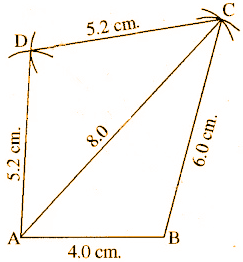Question 2: Construct a quadrilateral JUMP with JU = 3.5cm, UM = 4.0cm, MP = 5cm, PJ = 4.5cm, PU = 6.5cm.

Solution:

a. A line segment of length JU = 3.5 cm is drawn.

b. An arc of radius 6.5 cm from point U and an arc of radius 4.5 cm from point J which cuts the first arc at point P is drawn. Join UP and JP to obtain a triangle JUP.

c. Taking P as the centre, draw an arc of radius 5.0 cm and 4.0 cm from point U. Both arcs intersect each other at M.

d. PM and UM are joined.

The required quadrilateral JUMP is obtained.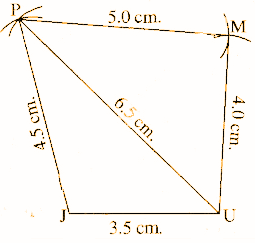Question 3: Construct a parallelogram MORE in which MO = 3.6cm, OR = 4.2cm, MR = 6.5cm. Measure the remaining sides and make a note of them.

Solution:

a. A line segment of length MO = 3.6 cm is drawn.

b. An arc of radius 4.2 cm from the point O and an arc of radius 6.5 cm from point M which intersect each other at R is drawn. Join MR and OR such that a triangle MOR is obtained.

c. An arc of radius 4.2 cm from point M and an arc of radius 3.6 cm from point R which intersect each other at E is drawn. Join ME and ER. The required parallelogram MORE is obtained. The measure of remaining sides is RE = 3.6 cm and ME = 4.2 cm.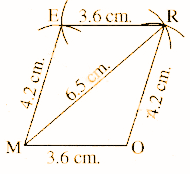Question 4: Construct a rhombus BEST in which BE = 4.5cm, ET = 6cm. Measure the length of the diagonal BS.

Solution:

a. A line segment of length BE = 4.5 cm is drawn.

b. An arc of radius 4.5 cm from point B and an arc of radius 6.5 cm from point E which intersect each other at T is drawn. Join BT and ET such that a triangle BET is obtained.

c. An arc of radius 4.5 cm from point T and an arc of radius 4.5cm from E which intersect each other at S is drawn. Join ES and TS. The required rhombus BEST is obtained. The diagonal BS is 6.8 cm.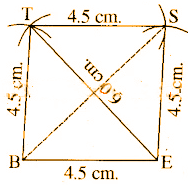Question 5: Construct a quadrilateral PQRS in which PQ = 4.4cm, QR = 4.0cm, RS = 6.4cm, SP = 2.8cm, QS = 6.6cm. Find the length of the diagonal PR.

Solution:

a. A line of segment PQ = 4.4 cm is drawn.

b. An arc of radius 2.8 cm from point P and an arc of radius 6.6 cm from point Q which intersect each other at point S is drawn. Join PS and QS such that a triangle PQS is obtained.

c. Taking S as the centre, an arc of radius 6.4 cm is drawn and taking Q as the centre, an arc of radius 4 cm is drawn which intersects each other at point R. Join RQ and RS.

The required quadrilateral PQRS is obtained. PR [diagonal length] = 6 cm.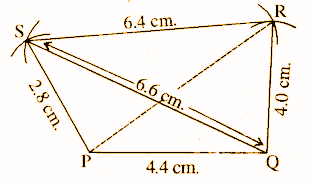### RBSE Maths Chapter 7: Exercise 7.2 Textbook Important Questions and Solutions

Question 1: Construct a quadrilateral LIFT in which LI = 4cm, IF = 3.0cm, TL = 2.5cm, LF = 4.5cm, IT = 4.0cm.

Solution:

a. A line segment LI = 4.0 cm is drawn.

b. An arc of radius 2.5 cm from point L and an arc of radius 4 cm from the point I which cut at point T is drawn. Join TL and TI such that a triangle LIT is obtained.

c. An arc of radius 4.5 cm from point L and an arc of radius 3.0cm from point I which intersect each other at F is drawn. Join LF and IF, TF.

The required quadrilateral LIFT is obtained.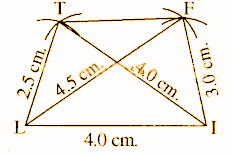Question 2: Construct a quadrilateral ABCD in which AB = 3.8cm, BC = 3.0cm, AD = 2.3cm, AC = 4.5cm, BD = 3.8cm. Measure the length of CD.

Solution:

a. A line segment AB = 3.8 cm is drawn.

b. An arc of radius 2.3 cm from point A and an arc of radius 3.8 cm from point B intersect each other at point D is drawn. Join AD and BD such that a triangle ABD is obtained.

c. An arc of radius 4.5 cm from point A and an arc of radius 3 cm from point B intersect each other at point C is drawn. Join AC and BC, CD.

The required quadrilateral ABCD is obtained.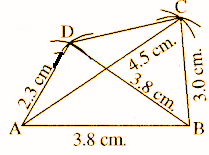Question 3: Construct a quadrilateral PQRS in which PS = 6.0cm, SR = 5.0cm, RQ = 7.5cm, PR = 6.0cm, SQ = 10.0cm.

Solution:

a. A line segment PQ = 7.5 cm is drawn.

b. An arc of radius 6 cm from point P and an arc of radius 10cm from point Q intersecting each other at S is drawn. Join PS and QS such that a triangle PQS is obtained.

c. An arc of 6 cm from point P and an arc of radius 7.5 cm from point Q intersecting each other at R is drawn. Join PR, QR and SR.

The required quadrilateral PQRS is obtained.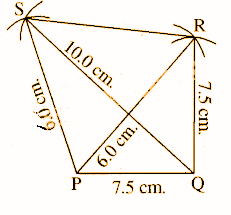Question 4: Construct a quadrilateral ABCD in which AB = BC = CD = 5.0cm and diagonal AC = 6.7cm and BD = 5.9cm.

Solution:

a. A line segment AB = 5 cm is drawn.

b. An arc of radius 6.7cm from point A and an arc of radius 5.0 cm from B intersecting each other at point C is drawn. Join AC and BC such that a triangle ABC is obtained.

c. From point B, an arc of radius 5.9 cm and from point C an arc of radius 5 cm is drawn. Both the arcs intersect each other at point D. Join BD and CD, AD.

The required quadrilateral ABCD is obtained.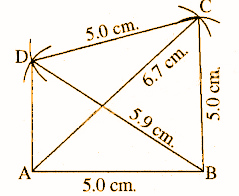Question 5: Construct a quadrilateral GOLD in which GO = 3.0cm, OL = 2.5cm, GD = 5.0cm, GL = 4cm, OD = 7cm.

Solution:

a. A line segment GO = 3.0 cm is drawn.

b. From point G, an arc of radius 4 cm and from point O, an arc of radius 2.5 cm intersecting each other at L is drawn. Join GL and OL such that a triangle GOL is obtained.

c. From point G, an arc of 5 cm and from point O, an arc of 7cm intersecting each other at D is drawn. Joint GD and OD, DL.

The required quadrilateral GOLD is obtained.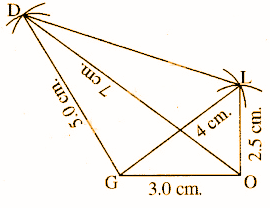### RBSE Maths Chapter 7: Exercise 7.3 Textbook Important Questions and Solutions

Question 1: Construct a quadrilateral ABCD in which AB = BC = 3.0cm, AD = CD = 5.0cm and ∠ABC = 120o.

Solution:

a. A line segment AB = 3.0 cm is drawn.

b. At point B with the help of pencil and compass, construct ABC = 120o with AB.

c. An arc of radius 5 cm from point B and an arc of radius 5 cm from point C intersecting each other at D is drawn. Join AD and CD. The required quadrilateral ABCD is obtained.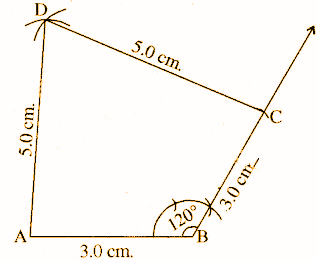Question 2: Construct a quadrilateral PQRS in which PQ = 2.8cm, QR = 3.1cm, RS = 2.6cm, SP = 3.3cm and ∠P = 60o.

Solution:

a. A line segment PQ = 2.8 cm is drawn.

b. At point P, an angle of QPS = 60° with PQ is constructed with the help of pencil and compass. An arc of radius 3.3 cm cuts PS from PX.

c. From point S, an arc of radius 2.6 cm and from point Q an arc of radius 3.1 cm intersecting each other at point R is drawn. Join SR and QR.

The required quadrilateral PQRS is obtained.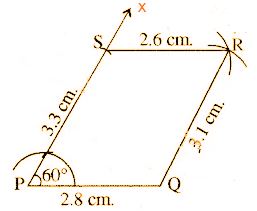Question 3: Construct a rectangle whose sides are 4.2cm and 2.5cm. Also, measure the diagonals.

Solution:

a. A line segment AB = 4.2 cm is drawn.

b. With the help of a pencil and compass, construct an angle BAX = 90° at point A with AB. An arc of 2.5 cm cuts from AX at D.

c. Similarly, construct, ABY = 90° with AB at point B and arc of 2.5 cm cuts a point C from BY.

d. Join CD.

The required rectangle ABCD is obtained with diagonals AC and BD = 4.9 cm.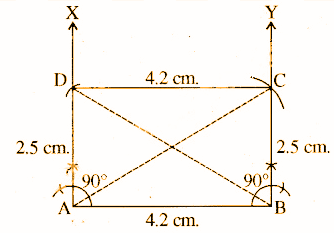Question 4: Construct a rhombus in which one angle is 75o and a side is 5.2cm.

Solution:

a. A line segment AB = 5.2 cm is drawn.

b. At point B, construct ABX = 75° with AB and cut an arc of 5.2 cm on BX. Mark the point as C.

c. An arc of radius 5.2 cm from C is drawn and an arc of radius 5.2 cm from A is cut. The point of intersection is marked as D.

d. Join AD and CD. The required rhombus is ABCD.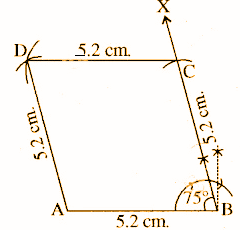Question 5: Draw a square with its side as 5.0cm.

Solution:

a. A line segment AB = 5.0 cm is drawn.

b. With the help of pencil and compass, construct an angle ABX = 90° at B.

c. B is taken as the centre and an arc of radius 5.0 cm is drawn such that it intersects BX at C.

d. Taking A as the centre, an arc of radius 5.0 cm and taking C as the centre, an arc of 5.0 cm is drawn intersecting each other at point D.

The required square ABCD is obtained.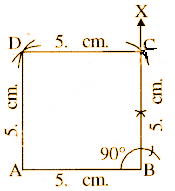### RBSE Maths Chapter 7: Exercise 7.4 Textbook Important Questions and Solutions

Question 1: Construct a quadrilateral KLMN in which KL = 4.8cm, LM = 5.0cm, MN = 3.8cm, ∠L = 72o and ∠M = 105o.

Solution:

a. A line segment KL = 4.8 cm is drawn.

b. At point L, construct angle KLX = 72°. An arc of 5.0 cm radius is cut on LX from point L. Mark the point as M.

c. At point M, construct angle LMN = 105° and cut an arc MN = 3.8 cm on MY. Mark the point as N.

d. Join KN.

Hence, KLMN is a required quadrilateral.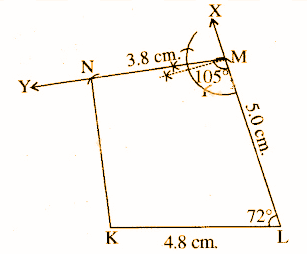Question 2: Construct a quadrilateral ABCD in which AB = BC = 3.0cm, AD = 5.0cm, ∠A = 90o and ∠B = 105o.

Solution:

a. A line segment AB = 3 cm is drawn.

b. At point A, construct angle BAX = 90o with AB and cut AD = 5.0 cm.

c. Similarly, construct angle ABY = 105°at point B with AB and an arc of 3 cm radius is drawn which cuts BY at C.

d. Join CD.

The required quadrilateral ABCD is obtained.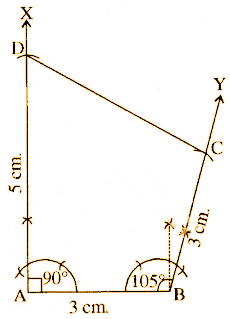Question 3: Construct a quadrilateral PQRS in which QR = 3.6cm, RS = 4.5cm, PS = 5.0cm, ∠R = 75o and ∠S = 120o.

Solution:

a. A line segment of line RS = 4.5 cm is drawn.

b. With the help of pencil and compass, construct an angle SRX = 75° at point R with SR and cut an arc of radius 3.6 cm from R on RX marking this point as Q.

c. Similarly, construct an angle RSY = 120° on RS, with the help of pencil and compass, from point SR, cut an arc of 5 cm radius marking this point as P.

d. Join PQ.

The required quadrilateral PQRS is obtained.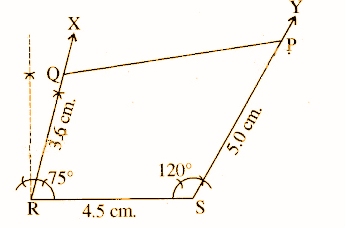Question 4: Construct a quadrilateral DEAR in which DE = 4.7cm, EA = 5.0cm, AR = 4.5cm, ∠E = 60o and ∠A = 90o.

Solution:

a. A line segment DE = 4.7 cm is drawn.

b. From E, a ray EX is drawn making an angle of 60° with DE from point E. An arc of 5.0 cm radius is cut on EX and marked as A.

c. Similarly, at point A, a ray AY is drawn making an angle of 90°. An arc of 4.5 cm radius is drawn on AY and marked as R.

d. Join DR.

The required quadrilateral DEAR is obtained.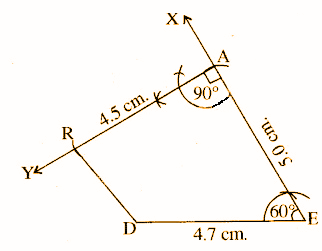Question 5: Construct a quadrilateral ABCD in which BC = 5.0cm, AB = 9.0cm, CD = 7.0cm, ∠B = 135o and ∠C = 90o.

Solution:

a. A line segment, AB = 9.0 cm is drawn.

b. Construct angle ABC = 135° at B. An arc of 5.0 cm radius is cut from BX. Mark this point as C.

c. At point C, construct an angle of 90° by a ray CY and cut an arc of 7.0 cm radius marking this point at D.

The required quadrilateral ABCD is obtained.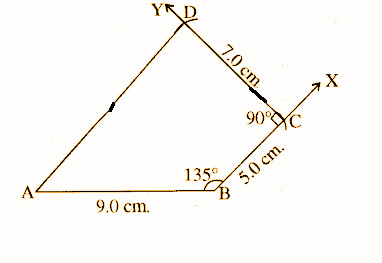### RBSE Maths Chapter 7: Exercise 7.5 Textbook Important Questions and Solutions

Question 1: Construct a quadrilateral MORE in which MO = 6cm, OR = 4.5cm, ∠M = 60o, ∠O = 105o, ∠R = 105o.

Solution:

a. A line segment MO = 6 cm is drawn.

b. At points, M and O construct the angles MOY = 105° and OMX = 60° with MO, respectively.

c. An arc of 4.5 cm radius is cut from point O on ray OY marking the point as R.

d. At point M, construct angle OME = 60° with OM intersecting MX at point E.

The required quadrilateral MORE is obtained.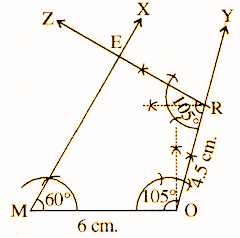Question 2: Construct a quadrilateral ABCD in which ∠B = 70o, ∠A = 54o, ∠D = 105o, AB = 6.2cm, AD = 5.7cm.

Solution:

a. A line segment AB = 6.2 cm is drawn.

b. From A, a ray AX is drawn making an angle of 54° with line segment AB. An arc of 5.7 cm radius is cut on AX and marked as point D.

c. From point D, making an angle of 105o a ray DZ is drawn.

d. From point B, a ray BY is drawn, making an angle of 70° cutting ray DZ at point C.

The required quadrilateral ABCD is obtained.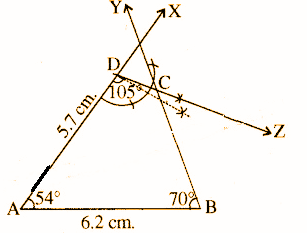Question 3: Construct a quadrilateral PQRS in which ∠P = 75o, ∠Q = 85o, ∠R = 110o, PQ = 4.1cm, QR = 3.9cm.

Solution:

a. A line segment PQ = 4.1 cm is drawn.

b. At point S, construct angle QPX = 75° with PQ.

c. At point Q, construct angle PQY = 85o with PQ and cut an arc QR = 3.9 cm from QY.

d. At point R, construct angle QRZ = 110° with QR intersecting PX at point S.

The required quadrilateral PQRS is obtained.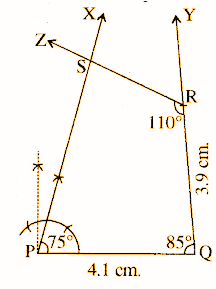Question 4: Construct a quadrilateral DNSI in which DN = 2.5cm, NS = 3.7cm, ∠I = 60o, ∠N = 120o, ∠S = 90o.

Solution:

The sum of all angles of a quadrilateral = 360°

∠D + ∠N + ∠S + ∠I = 360°

∠D = 360° – (120o + 90o + 60°)

= 360° – 270°

= 90o

a. A line segment NS = 3.7 cm is drawn.

b. At points, N and S construct angle SNX =120° and NSY = 90° with NS.

c. An arc is cut with DN = 2.5 cm radius from NX.

d. At point D, construct angle NDZ= 90° with DN and intersecting SY at I.

The required quadrilateral DNSI is obtained.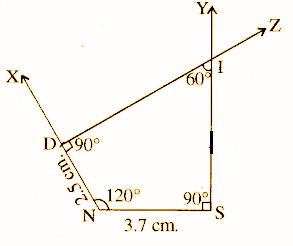### RBSE Maths Chapter 7: Exercise 7.6 Textbook Important Questions and Solutions

Question 1: Construct a square in which

[i] Perimeter is 20cm.

[ii] Sum of two adjacent sides is 9cm.

Solution:

[i] The perimeter of the given square = 20cm.

Side of square = Perimeter / 4 = [20 / 4] = 5cm [each side of the square]

Each angle in the square is 90o.

a. A line segment AB = 5 cm is drawn.

b. At points, A and B, construct angle BAX = 90° and ABY = 90° with AB.

c. Ray AX cuts AD = 5 cm and ray BY cuts BC = 5 cm.

d. Join CD.

The required square ABCD is obtained.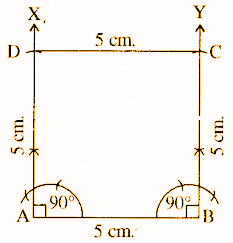[ii] The sum of two adjacent sides is 9cm.

Each side of square = 9 / 2 = 4.5cm

a. A line segment AB = 4.5cm is drawn.

b. At points, A and B construct angles BAX = 90° and ABY = 90° with AB, respectively.

c. From point B, an angle of 90° is made and an arc of 4.5 cm is cut marking this point as C.

d. Join C and D. The required square ABCD is obtained.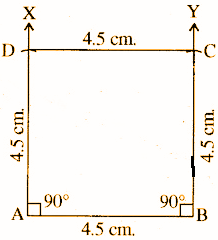Question 2: Construct a square PQRS in which diagonals meet at point O and PO = 2.8cm.

Solution:

The diagonals of a square are equal and bisect each other.

PO = 2.8cm = 2 * 2.8 = 5.6cm

a. A line segment of diagonal PR = 5.6 cm is drawn.

b. A perpendicular bisector XY of PR which intersects at point O is drawn.

c. Taking O as the centre, an arc of radius 2.8 cm is drawn on both sides of PR which cuts the perpendicular bisector XY at Q and S.

d. PQ, QR, RS and SP are joined.

The required square PQRS is obtained.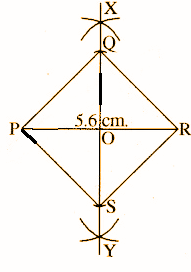Question 3: Construct a rectangle in which its adjacent sides are 8cm and 6cm.

Solution:

a. A line segment AB = 8 cm is drawn.

b. At point A construct angle BAX = 90° with AB.

c. An arc of 6.0 cm radius is cut on AX marking this point as D.

d. At point B construct ABY = 90° with AB and cut BC = 6 cm from ray BY at C.

e. Join CD.

The required rectangle ABCD is obtained.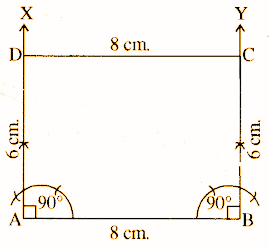Question 4: Construct a rectangle PQRS in which PQ = 5.5cm and the diagonal QS = 6.2cm.

Solution:

In the case of a rectangle, the opposite sides are parallel and equal to each other, the diagonals are equal to each other and each angle is 90o.

a. A line segment PQ = 5.5 cm is drawn.

b. At points P and Q construct angle QPX = 90o and PQY = 90o with PQ, respectively.

c. At point P, an arc of radius 6.2 cm which intersects the ray QY at point R is drawn. Join PR.

d. At point Q, an arc of radius 6.2 cm which intersects ray PX at S. Join QS and SR.

The required rectangle PQRS is obtained.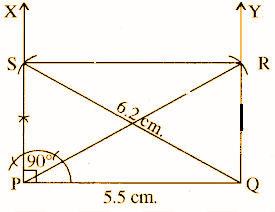Question 5: Construct a rectangle EFGH while EF = 4.0cm and diagonal EG = 5.0cm.

Solution:

a. A line segment EF = 4.0 cm is drawn.

b. At point E and F construct angle, FEX = 90° and EFY = 90° with EF respectively using ruler and compass.

c. An arc of 5 cm radius from point E is cut on FY marking the point as G.

d. Also, an arc of 5 cm is cut on EX marking the point as H.

e. EG, FG and GH are joined.

The required rectangle EFGH is obtained.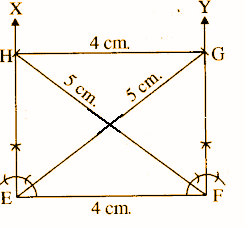Question 6: Construct a rhombus DEFG in which FG = 4.8cm and diagonal EG = 3.4cm

Solution:

a. A diagonal EG = 3.4 cm is drawn.

b. At point E and G, two arcs of radius 4.8 cm and 4.8 cm are drawn on both sides of EG intersecting each other at point D and F, respectively.

d. ED, GD, GF and EF are joined.

The required rhombus DEFG is obtained.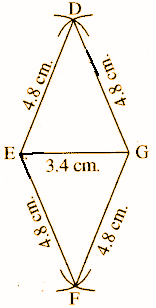Question 7: Construct a rhombus ABCD in which BC = 4.0cm and B = 75o.

Solution:

A rhombus is a special case of a parallelogram.

∠B + ∠C = 180o

∠C = 180o – 75o = 105o

a. A line BC = 4 cm is drawn.

b. At points B and C, construct angles CBX = 75° and BCY =105o with BC using ruler and compass.

c. From points B and C, two arcs each of radius 4 cm are cut on BX and CY marking the points A and D.

The required rhombus ABCD is obtained.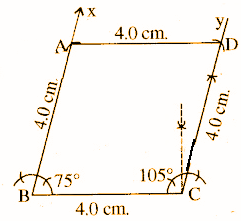### RBSE Maths Chapter 7: Additional Questions and Solutions

Question 1: Discuss any two necessary conditions for the construction of a unique square.

Solution:

A quadrilateral can be constructed if the following values are given.

[i] The measure of the length of its four sides and a diagonal is to be known.

[ii] The measure of the two diagonals and the three sides are known.

Question 2. Define a square.

Solution:

A square is a rectangle with equal sides.

Question 3: If the measure of the two diagonals of a parallelogram is equal, then mention the type of quadrilateral.

Solution:

Rectangle

Question 4: Define a rhombus.

Solution:

A parallelogram with each of the sides having equal measure is a rhombus.

Question 5. Define a trapezium.

Solution:

A quadrilateral with a pair of parallel sides is a trapezium.

Question 6: Write the properties of a parallelogram.

Solution:

• The opposite sides are equal.

• The opposite angles are equal.

• The diagonals bisect each other.

Question 7: Discuss any two conditions for constructing a unique quadrilateral.

Solution:

A unique quadrilateral can be constructed

• If the length of its four sides and a diagonal is known.

• If its two adjacent sides and three angles are known.

Question 8: Can one draw a parallelogram BATS where BA = 5 cm, AT= 6 cm and AS = 6.5 cm? Why?

Solution:

Yes, the parallelogram BATS can be drawn with the given data as it can be divided into two triangles BAS and SAT.

Question 9: Can one draw a rhombus ZEAL where ZE = 3.5 cm, diagonal EL = 5 cm? Why?

Solution:

Yes, the rhombus ZEAL can be drawn with the given data as it can be divided into two triangles ZEL and LEA.